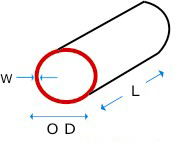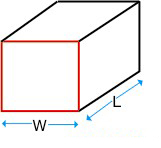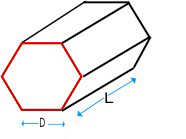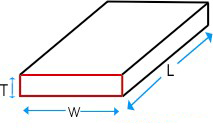## Formulas

Product Calculator

## Formulas

 1. Formula for Calculating S. S. Pipe Weight : 0.D. (mm) - W. Thick (mm) X W. Thick (mm) X 0.0248 = Wt. Per Mtr. 0.D. (mm) - W. Thick (mm) X W. Thick (mm) X 0.00756 = Wt. Per Feet2. Formula for Calculating S. S. Bar Weight : DIA (mm) X DIA (mm) X 0.00623 = Wt. Per Mtr. DIA (mm) X DIA (mm) X 0.0019 = Wt. Per Feet 3. Formula for Calculating S. S. Square Bar Weight : DIA (mm) X DIA (mm) X 0.00788 = Wt. Per Mtr. DIA (mm) X DIA (mm) X 0.0024 = Wt. Per Feet4. Formula for Calculating S. S. Hexagonal Bar Weight: DIA (mm) X DIA (mm) X 0.00680 = Wt. Per Mtr. DIA (mm) X DIA (mm) X 0.002072 = Wt. Per Fee5. Formula for Calculating S.S. Flat Bar Weight: Width (mm) X Thick (mm) X 0.00798 = Wt. Per Mtr. Width (mm) X Thick (mm) X 0.00243 = Wt. Per Feet 6. Formula for Calculating S.S. Sheet & Plates Weight: Length (mtrs) X Width (mtrs) X Thick (mm) X 8.068 = Wt. Per PC. Length (feet) X Width (feet) X Thick (mm) X 3/4 = Wt. Per PC7. Formula for Calculating S.S. Circle Weight: Dia (mm) X Dia (mm) X Thick (mm) ÷ 160 = Gms. Per PC Dia (mm) X Dia (mm) X Thick (mm) X 0.0000063 = Wt. Per PC 8. Conversion of Mtrs to Feet: Weight of 1 Mtrs ÷  3.2808  = Weight Per Feet